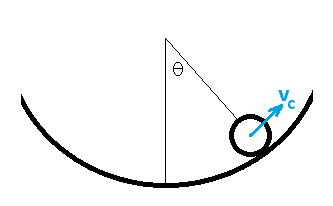# Marble rolling on ramp harmonic motion

## Homework Statement

A perfectly solid marble of radius R rolls without slipping at the bottom of a spherical bowl of a radius 6R. The marble rolls back and forth in the vertical plane executing simple harmonic motion close to the lowest point. How long does it take the marble to go down one side and up the other from its maximum height to its maximum height?

a) Cannot be determined without knowing amplitude
b) ##\pi \sqrt{\frac{7R}{g}}##
c) ##\pi \sqrt{\frac{7R}{2g}}##
d) ##\pi \sqrt{\frac{7R}{5g}}##
e) ##\pi \sqrt{\frac{5R}{7g}}##

## Homework Equations

##\tau_{net} = I\alpha##
##I = 2/5 mR^2## (for sphere)
##F = ma##
##\tau = rFsin\theta##

## The Attempt at a Solution

I got answer choice d, but the key says b is correct.
I set up ##\tau_{net} = I\alpha = -fR## where ##f## is the static friction force. I believe gravity does not contribute to the torque, since it acts at the center-of-mass; neither does the normal force since it is always antiparallel to the radius vector from the center of the marble to the point of application. That only leaves the static friction force.
Then, I know that ##F = ma = -mgsin\theta + f \approx -mg\theta + f## tangent to the bowl, using the sine approximation for small theta.
Plugging back into torque net, I get that ##(2/5 mR^2)\alpha = -(ma + mg\theta)R##. Divide by an ##mR## and simplify to get ##7/5 R\alpha = -g\theta##. This means that ##\omega = \sqrt{\frac{5g}{7R}}##. Finally I realize that the desired time is half the period and ##T = 2\pi / \omega## so the desired time is ##\pi \sqrt{\frac{7R}{5g}}##, or at least that's what I got.

Where did I go wrong?

## Answers and Replies

TSny
Homework Helper
Gold Member
##7/5 R\alpha = -g\theta##.
OK up to here!

Hint: ##\alpha \neq \ddot \theta##.
##\dot \theta## is not the rate of spin of the ball about its center.
Consider the kinematics more carefully. What is the meaning of the angle ##\theta##?

OK up to here!

Hint: ##\alpha \neq \ddot \theta##.
##\dot \theta## is not the rate of spin of the ball about its center.
Consider the kinematics more carefully. What is the meaning of the angle ##\theta##?
Hmm.. I think what you're trying to get at is that ##\alpha## isn't directly related to the change in the angle wrt the center of the bowl; rather, it refers to the rotation of the ball. So that means ##\alpha = 5\ddot \theta##?

TSny
Homework Helper
Gold Member
Hmm.. I think what you're trying to get at is that ##\alpha## isn't directly related to the change in the angle wrt the center of the bowl; rather, it refers to the rotation of the ball. So that means ##\alpha = 5\ddot \theta##?
Yes. Can you derive the relation ##\alpha = 5\ddot \theta##?

Yes. Can you derive the relation ##\alpha = 5\ddot \theta##?
I made a guess there by thinking about how if the ball rolled through an angle ##\phi## wrt its center of mass, it would trace out an arc ##R\phi##. But then, wrt the center of the bowl, if the angle changed by that same amount the center-of-mass of the marble would trace out an arc ##(6R - R) \phi##. And then I assume this relation holds for the angular accelerations. I'm not sure how to do it rigorously.

EDIT: I think since ##\alpha = a/r## in general, then wrt to the ball's COM ##\alpha = a/R## but wrt to the center of the bowl ##\alpha' = \ddot \theta = a/(5R)##. So ##\alpha = 5\ddot \theta##.

Last edited:
TSny
Homework Helper
Gold Member
I made a guess there by thinking about how if the ball rolled through an angle ##\phi## wrt its center of mass, it would trace out an arc ##R\phi##. But then, wrt the center of the bowl, if the angle changed by that same amount the center-of-mass of the marble would trace out an arc ##(6R - R) \phi##. And then I assume this relation holds for the angular accelerations. I'm not sure how to do it rigorously.
Yes, that's good. Another way is to consider the velocity of the center of the ball ##v_c##. Let ##\phi## represent the angle of rotation of the ball about its center. Write ##v_c## in two different ways: (1) in terms of ##\dot \theta## and (2) in terms of ##\dot \phi##.#### Attachments

Yes, that's good. Another way is to consider the velocity of the center of the ball ##v_c##. Let ##\phi## represent the angle of rotation of the ball about its center. Write ##v_c## in two different ways: (1) in terms of ##\dot \theta## and (2) in terms of ##\dot \phi##.
View attachment 218891
##v_c = 5R\dot \theta = R\dot \phi##.

TSny
Homework Helper
Gold Member
##v_c = 5R\dot \theta = R\dot \phi##.
Yes. I guess that's pretty much the same as your first derivation in terms of arc lengths.

TSny
Homework Helper
Gold Member
EDIT: I think since ##\alpha = a/r## in general, then wrt to the ball's COM ##\alpha = a/R## but wrt to the center of the bowl ##\alpha' = \ddot \theta = a/(5R)##. So ##\alpha = 5\ddot \theta##.
I think you nailed it!I think you nailed it!Thank you!